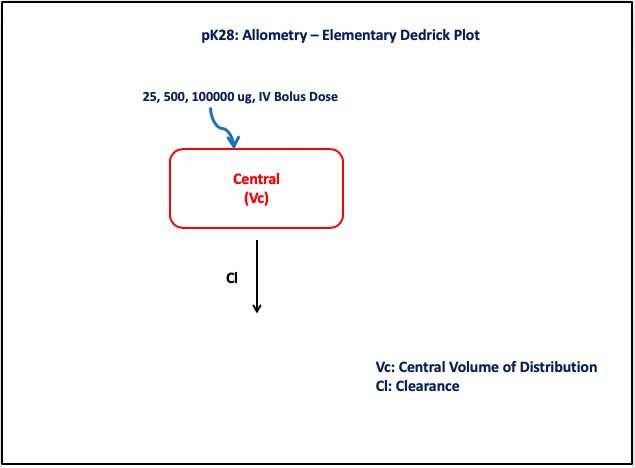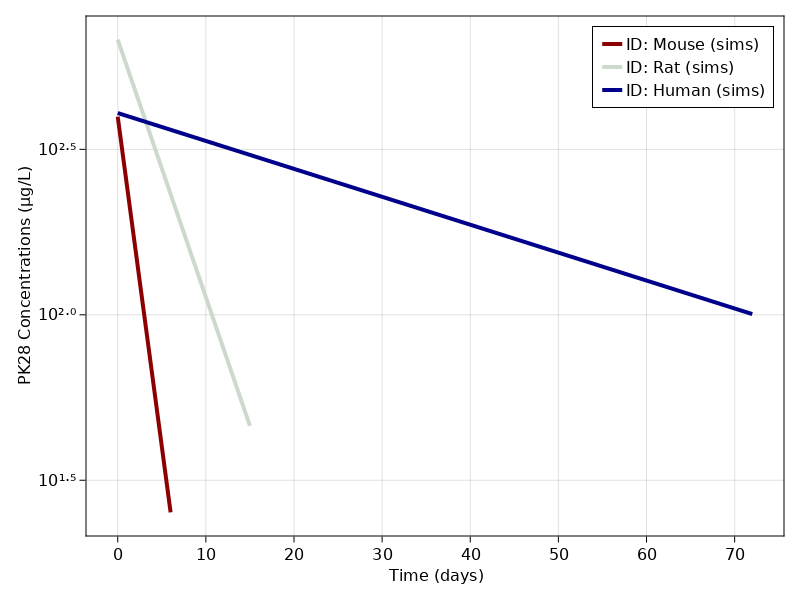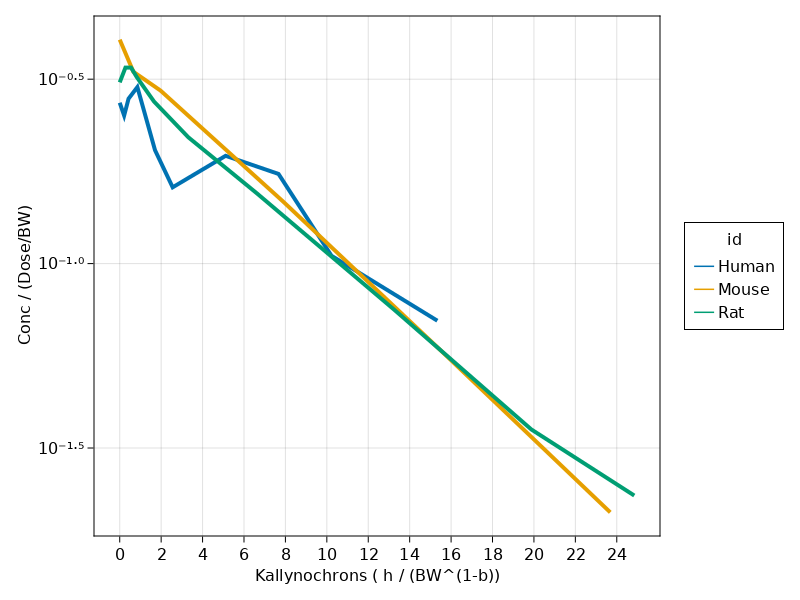# Exercise PK28 - Allometry - Elementary Dedrick Plot

### Background

• Structural model - One Compartment Model with Linear Elimination

• Route of administration - IV-Bolus

• Dosage Regimen - 25, 500, 100000 μg administered after complete washout

• Number of Subjects - 3 species (Mouse, Rat, Human)### Learning Outcome

• To learn about Allometry - Elementary Dedrick Plots

• To simultaneously simulate an allometric model to concentration-time data obtained from three different species

### Libraries

Call the "necessary" libraries to get started

using Random
using Pumas
using PumasUtilities
using CairoMakie
using DataFramesMeta
using AlgebraOfGraphics


### Model

The given data follows a One-Compartment Model with linear elimination

pk_28       = @model begin
desc    = "One Compartment Model"
timeu   = u"hr"
end

@param begin
"Clearance (L/hr)"
a       ∈ RealDomain(lower=0)
"Scaling parameter for Cl"
b       ∈ RealDomain(lower=0)
"Volume of Distribution (L)"
c       ∈ RealDomain(lower=0)
"Scaling parameter for Vc"
d       ∈ RealDomain(lower=0)
Ω       ∈ PDiagDomain(2)
"proportional RUV"
σ²_prop ∈ RealDomain(lower=0)
end

@random begin
η       ~ MvNormal(Ω)
end

@covariates BW

@pre begin
Cl      = a * (BW)^b * exp(η)
Vc      = c * (BW)^d * exp(η)
end

@dynamics begin
Central' = -(Cl/Vc)*Central
end

@derived begin
cp      = @. Central/Vc
"""
Observed Concentration (ug/L)
"""
dv      ~ @. Normal(cp, sqrt(cp^2*σ²_prop))
end

@observed begin
conc = Central / Vc
nca    := @nca conc
AUC     = NCA.auc(nca)
CL      = NCA.cl(nca)
V       = NCA.vz(nca)
t_half  = NCA.thalf(nca)
end
end

PumasModel
Parameters: a, b, c, d, Ω, σ²_prop
Random effects: η
Covariates: BW
Dynamical variables: Central
Derived: cp, dv
Observed: cp, dv, conc, AUC, CL, V, t_half


### Parameters

• a - typical value of clearance among the species

• b - scaling parameter for Cl

• c - typical value of volume of distribution among the species

• d - scaling parameter for Vc

param = ( a       = 0.319142230070251,
b       = 0.636711976371785,
c       = 3.07665859278123,
d       = 1.03093780182922,
Ω       = Diagonal([0.0,0.0]),
σ²_prop = 0.01)

(a = 0.319142230070251, b = 0.636711976371785, c = 3.07665859278123, d = 1.
03093780182922, Ω = [0.0 0.0; 0.0 0.0], σ²_prop = 0.01)


### Dosage Regimen

• Species 1 (Mouse) - 25μg IV-Bolus and bodyweight (23grams)

• Species 2 (Rat) - 500μg IV-Bolus and bodyweight (250grams)

• Species 3 (Human) - 100000μg IV-Bolus and bodyweight (70kg)

ev1      = DosageRegimen(25, cmt = 1, time = 0, route = NCA.IVBolus)
sub1     = Subject(id="Mouse", events = ev1, covariates = (BW = 0.023, dose = 25), time = [0,0.167,0.5,2,4,6])
ev2      = DosageRegimen(500, cmt = 1, time = 0,route = NCA.IVBolus)
sub2     = Subject(id="Rat", events = ev2, covariates = (BW = 0.250, dose = 500), time = [0,0.167,0.33,0.5,1,2,4,8,12,15])
ev3      = DosageRegimen(100000, cmt = 1, time = 0, route = NCA.IVBolus)
sub3     = Subject(id="Human", events = ev3, covariates = (BW = 70, dose = 100000), time = [0,1,2,4,8,12,24,36,48,72])
pop3_sub = [sub1,sub2,sub3]

Population
Subjects: 3
Covariates: BW, dose
Observations:


### Simulation

Random.seed!(123)
sim_pop3 = simobs(pk_28, pop3_sub, param)


### Visualization

A plot of Concentration vs Time data from three different species

f, a, p = sim_plot(pk_28, sim_pop3,
observations = :cp,
color = :redsblues,
linewidth = 4,
axis = (xlabel = "Time (days)",
ylabel = "PK28 Concentrations (μg/L)",
xticks = 0:10:70, yscale = log10))
axislegend(a)
fAn Elementary-Dedrick Plot of dose and bodyweight normalized plasma concentration vs bodyweight normalized time

pk28df = @chain sim_pop3 begin
DataFrame
@rtransform @passmissing :dv = round(:dv, sigdigits = 2)
@rtransform @passmissing :amt_bw  = :dose / :BW
@rtransform @passmissing :yfactor = :dv / :amt_bw
@rtransform @passmissing :bw_b    = :BW^(1-0.636)
@rtransform @passmissing  :xfactor = :time / :bw_b
dropmissing!(:dv)
end

plt = data(pk28df) *
mapping(:xfactor => "Kallynochrons ( h / (BW^(1-b))",
:yfactor => "Conc / (Dose/BW)", color = :id) *
visual(Lines, linewidth = 4)
draw(plt, axis = (; yscale = log10, xticks = 0:2:24))